### Group Theory

In the last quiz, we looked at symmetries of shapes, which are rigid transformations that send the shape to itself. At the end of the quiz, we saw that we could combine symmetries by doing one after the other, and thus form a kind of “multiplication” on the symmetries.

In this quiz, we’ll explore this idea further, and in the next quiz, this will lead to the definition of a group.

To start, consider this equilateral triangle: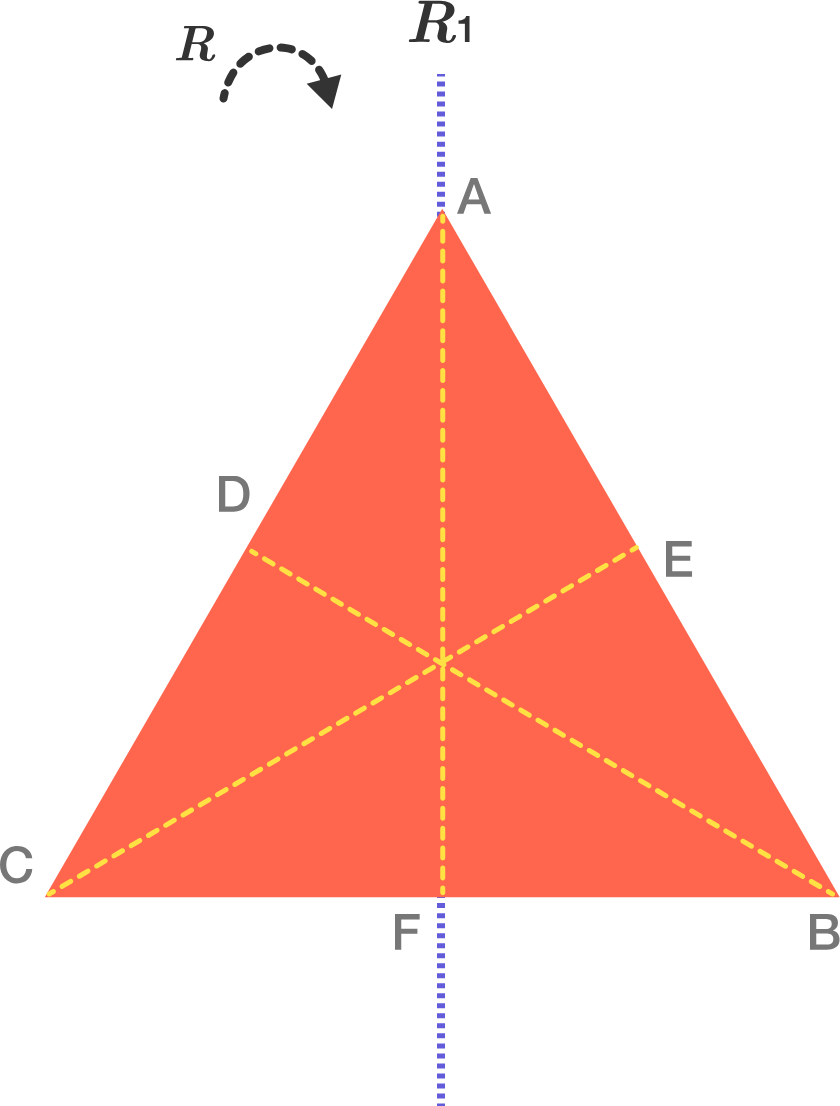Let $R$ be clockwise rotation by $120^{\circ},$ and let $R_1$ be a reflection about the line segment $\overline{AF}.$ What symmetry do you get when you apply $R_1$ followed by $R ?$

Hint: Investigate where the three vertices of the triangle are sent by $R_1$ followed by $R.$

Note: The rotations are all about the point where the dashed lines meet.

# Combining Symmetries

Now let’s combine two reflections.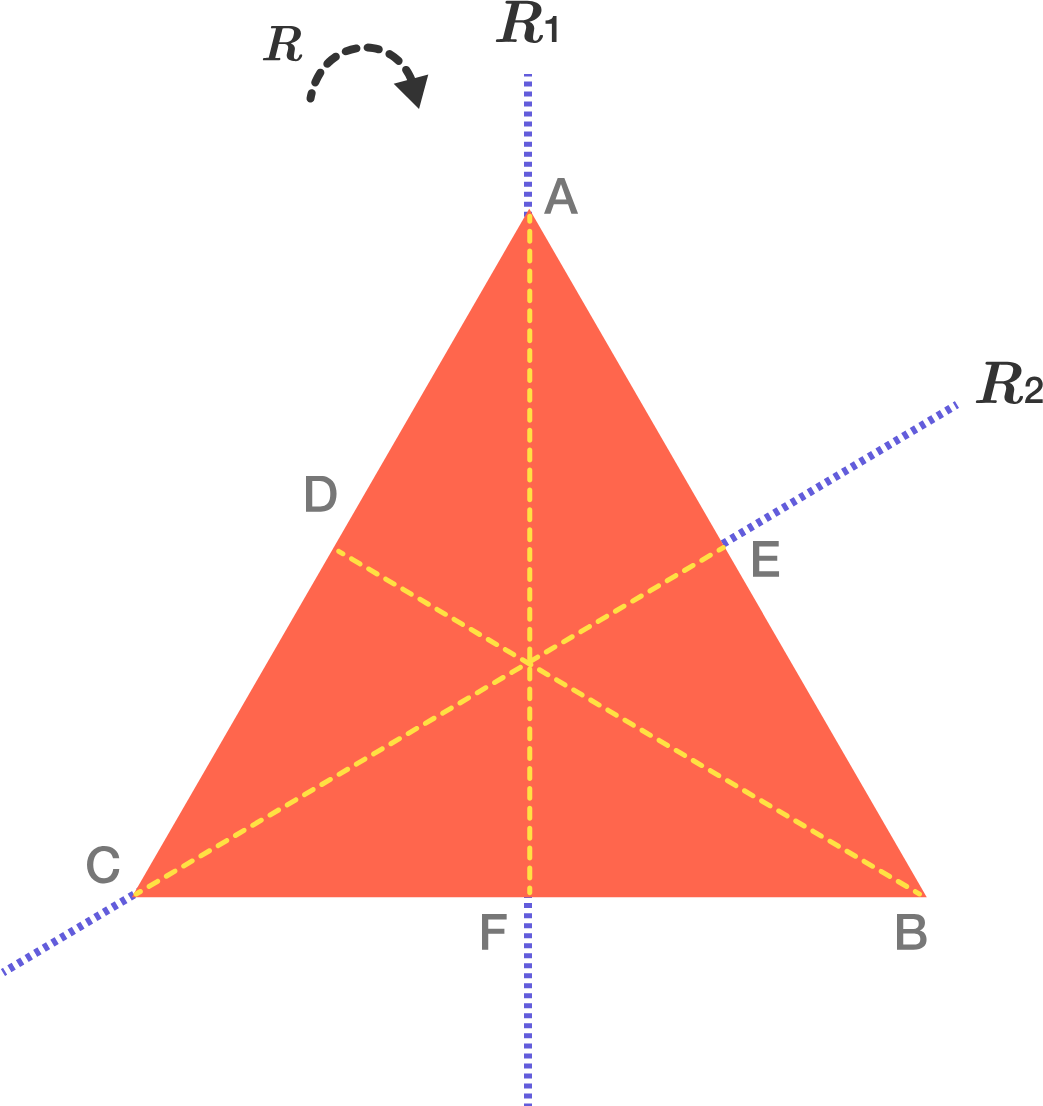Let $R_1$ be reflection about the line $\overline{AF},$ and let $R_2$ be reflection about the line $\overline{CE}.$ What symmetry do you get by applying first $R_1$ and then $R_2$?

Note: After the lines of reflection are set they do not change, even if the points they were originally based on change position.

# Combining Symmetries

In fact, we can combine any two symmetries of a shape by doing one after the other, and obtain a third symmetry of the shape. We express this with the following notation: If $A$ and $B$ are symmetries of a shape, $A * B$, or sometimes just $AB,$ denotes the symmetry you get by applying $B$ first, and then $A.$ We will call this new symmetry the product of $A$ and $B.$

For example, in the previous problem, if we let $\phi_1$ denote the reflection about $\overline{AF},$ let $\phi_2$ denote the reflection about $\overline{CE},$ and let $R$ denote clockwise rotation by $120^{\circ},$ then we can write the equation $\phi_2 * \phi_1 = R.$ To really understand the symmetries of an object, we should know not only how many symmetries there are, but what the rules are for how they combine with each other. For the equilateral triangle, we could make a 6 x 6 “multiplication table” that tells you, for any two of the six symmetries of the triangle, what their product is. In the next problems, we will do exactly that for a slightly simpler object.

# Combining Symmetries

In the next few problems, we will work out the symmetry multiplication table for the letter I. For starters, how many symmetries does this letter have? (Remember to include the identity symmetry.)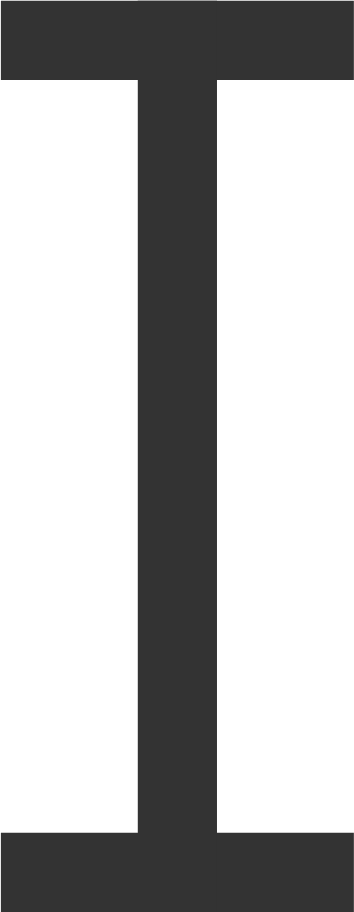# Combining Symmetries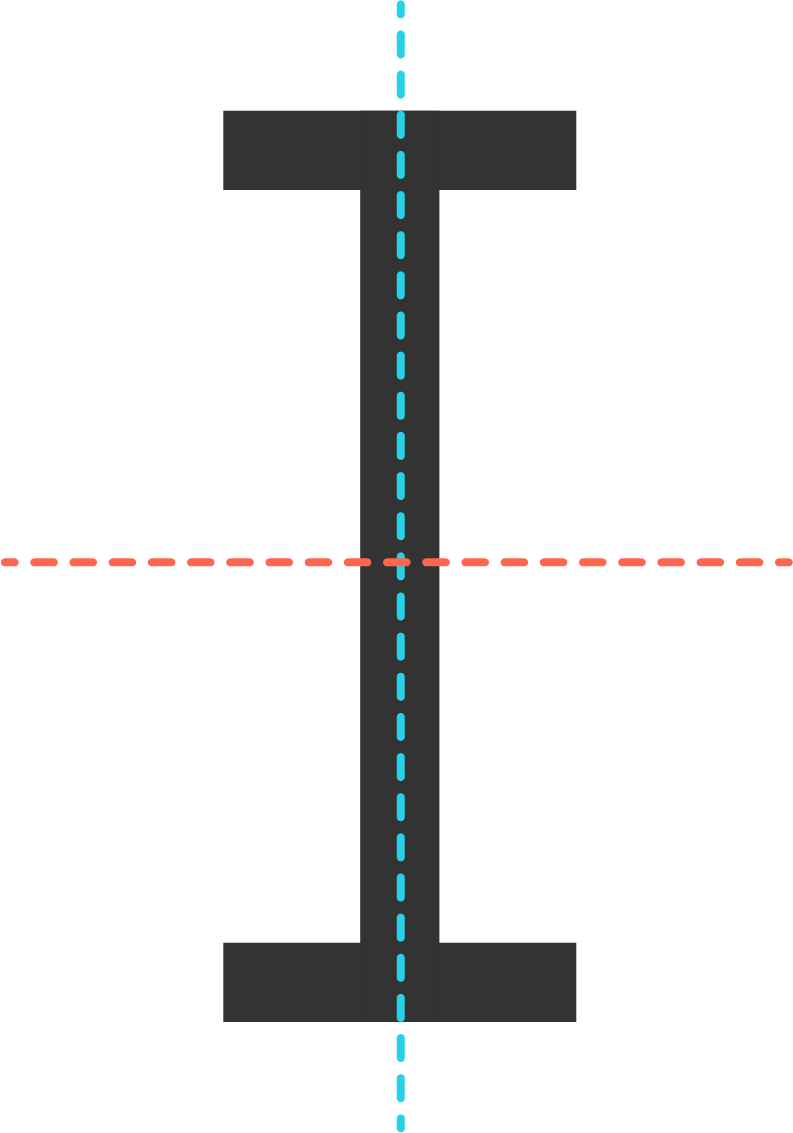We’ve seen that the letter I has four symmetries: A horizontal reflection (flipping across the blue line) $H;$ a vertical reflection (flipping across the red line) $V;$ rotation by $180^{\circ}$ $R;$ and the identity transformation $I$.

Let’s start working out the 4 x 4 multiplication table for this object!

What are $H * H,$ $V * V,$ and $R * R?$

# Combining Symmetries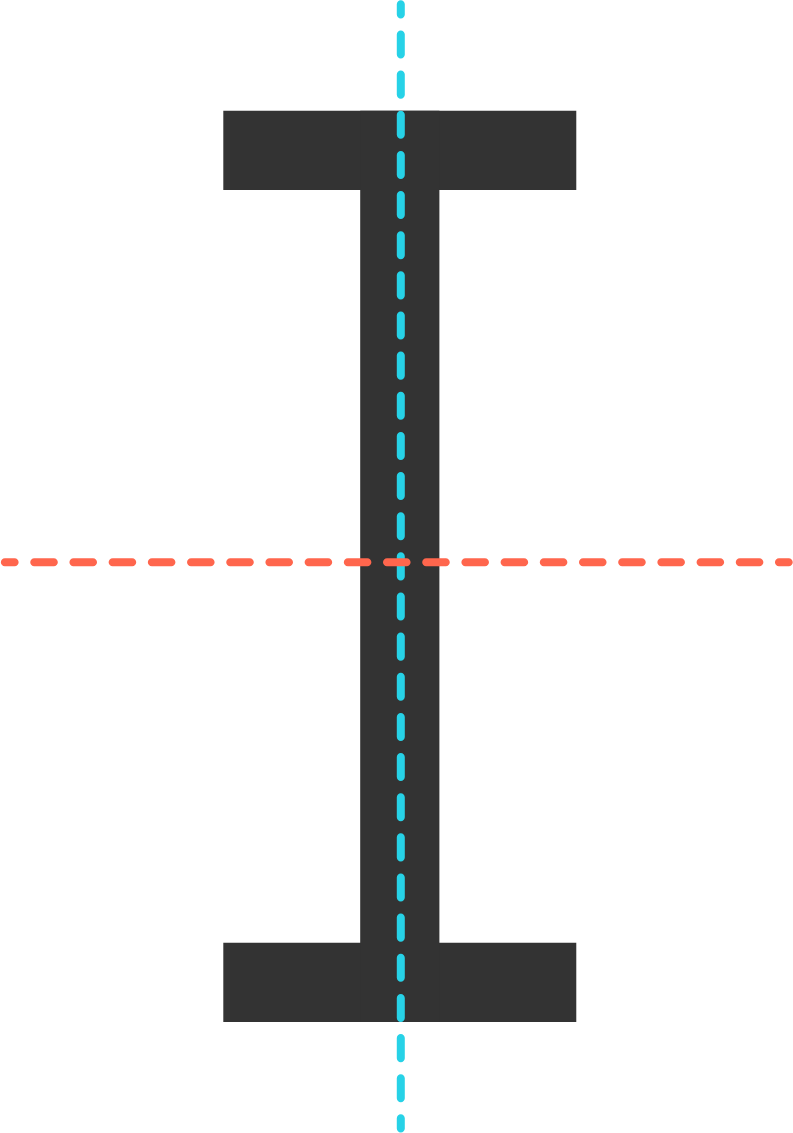So far, our multiplication table for the symmetries of the letter I looks like this: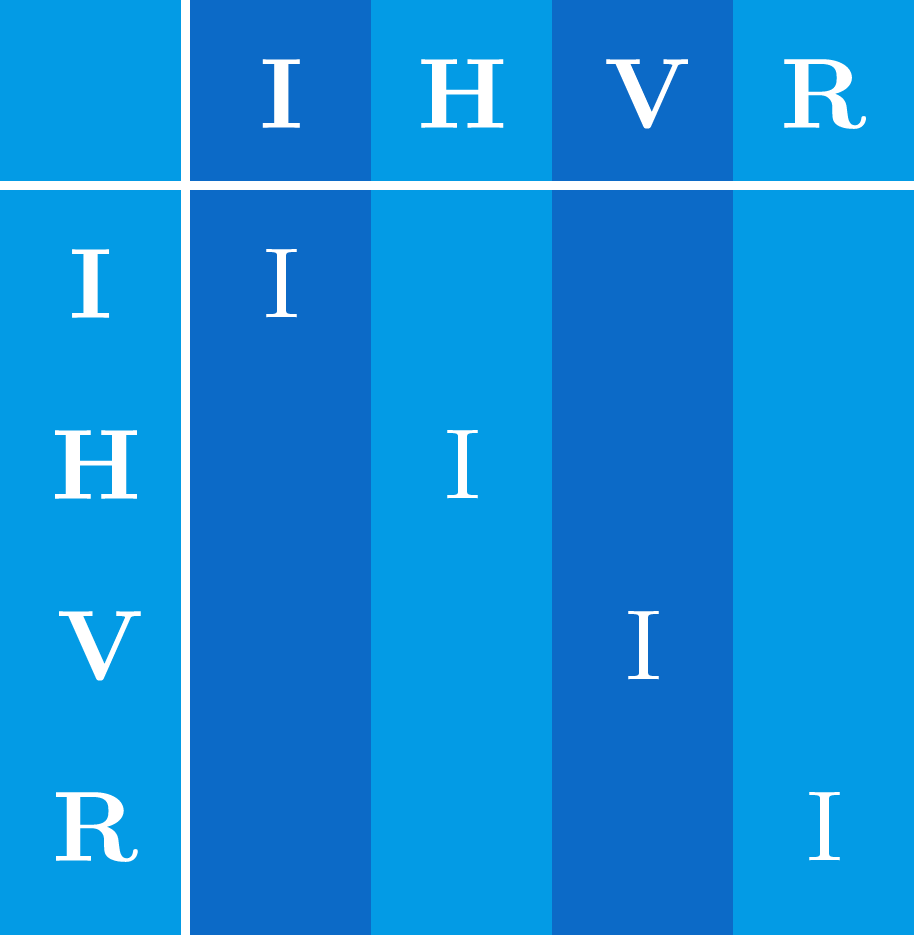This table, once it’s filled out, will be used to read off the product of symmetries. For instance, $H * V$ will appear in the row labeled $H$ and the column labeled $V,$ although that entry hasn’t been filled out yet. We filled out the diagonals with $I$ because we saw in the last problem that $H * H,$ $V * V$ and $R * R$ are all $I$.

Let’s fill out the first row and column of the table. The first row has products like $I * H$ and $I * V,$ which result from doing a transformation like $H$ or $V$ and then doing the identity. (Remember a product like $I * H$ means to do $H$ first.) The first column are products resulting from doing the identity first, and then some other transformation.

Which of the following correctly fills out the first row and column?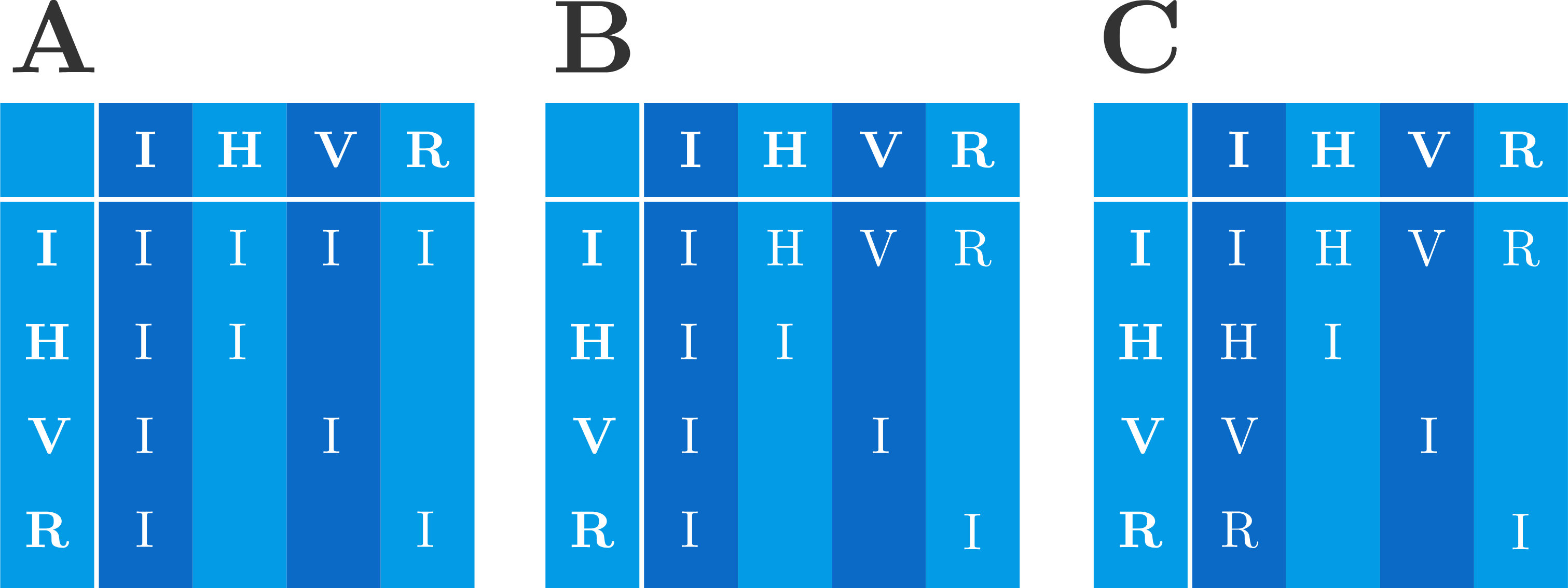# Combining SymmetriesNow for some more interesting combinations! What are $H * V$ and $V * H?$ (Hint: When we worked out the triangle, it was useful to see where the vertices went under the product. Here, it’s useful to see where the four “outer corners” of the letter I go.)

# Combining SymmetriesWe’re almost done! So far we have the following table: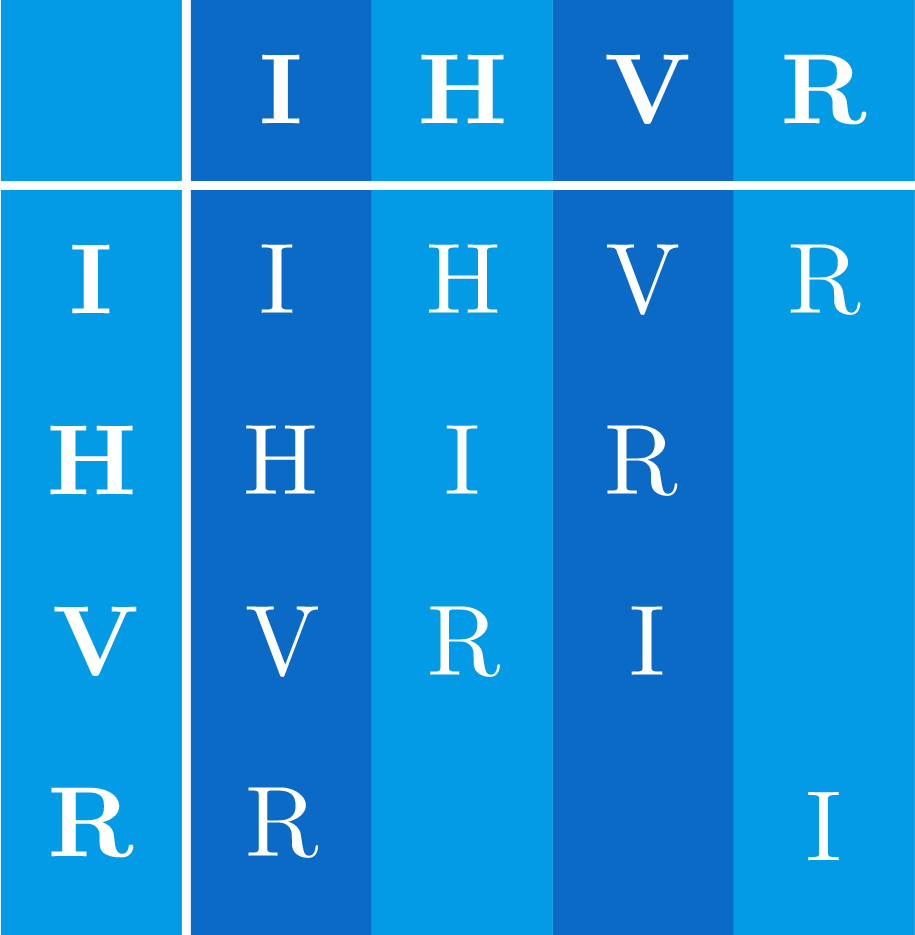Fill out the remaining four spaces by working through the remaining products, $H * R,$ $V * R,$ etc.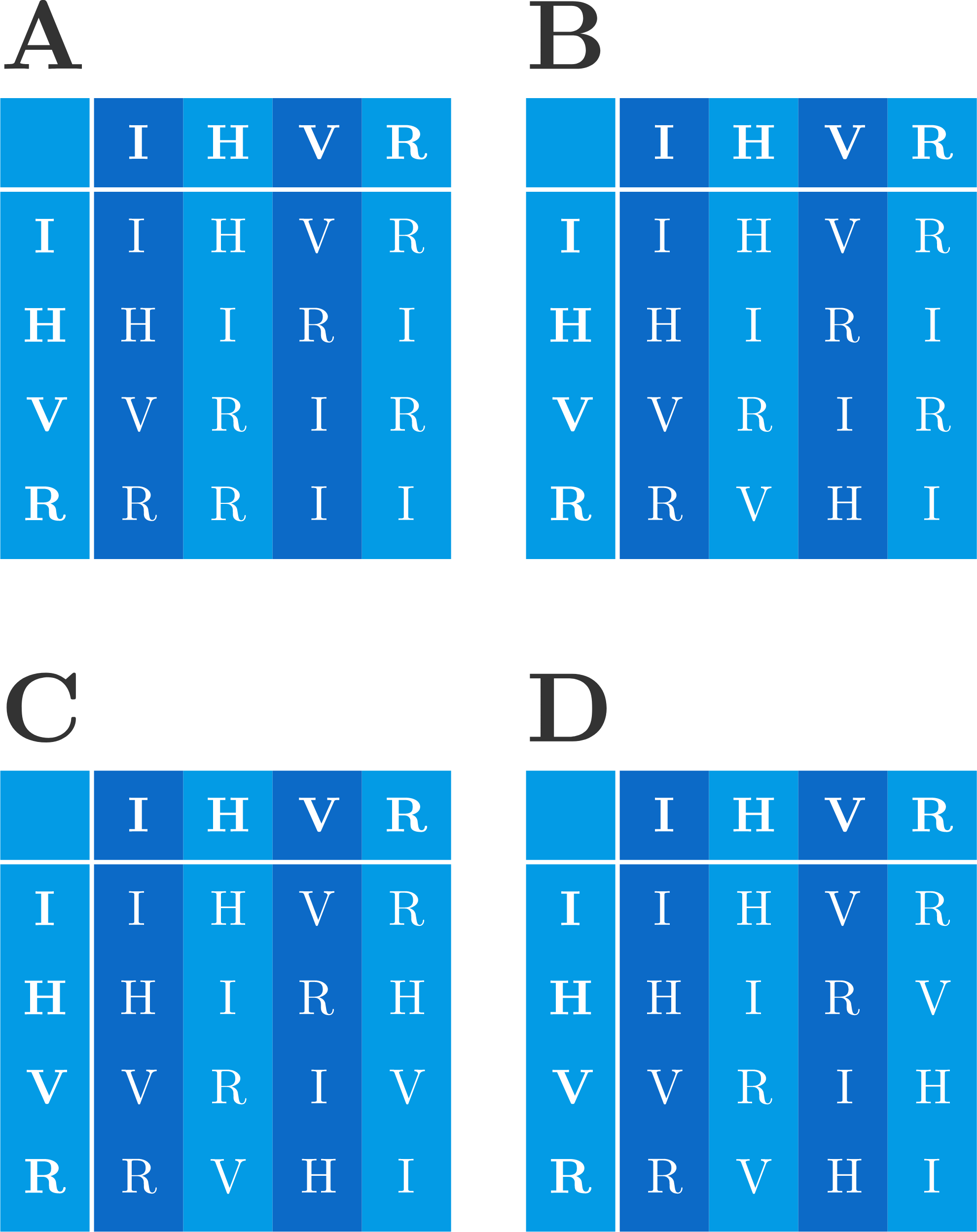# Combining Symmetries

We’ve made our first multiplication table--or what we will soon call a group table: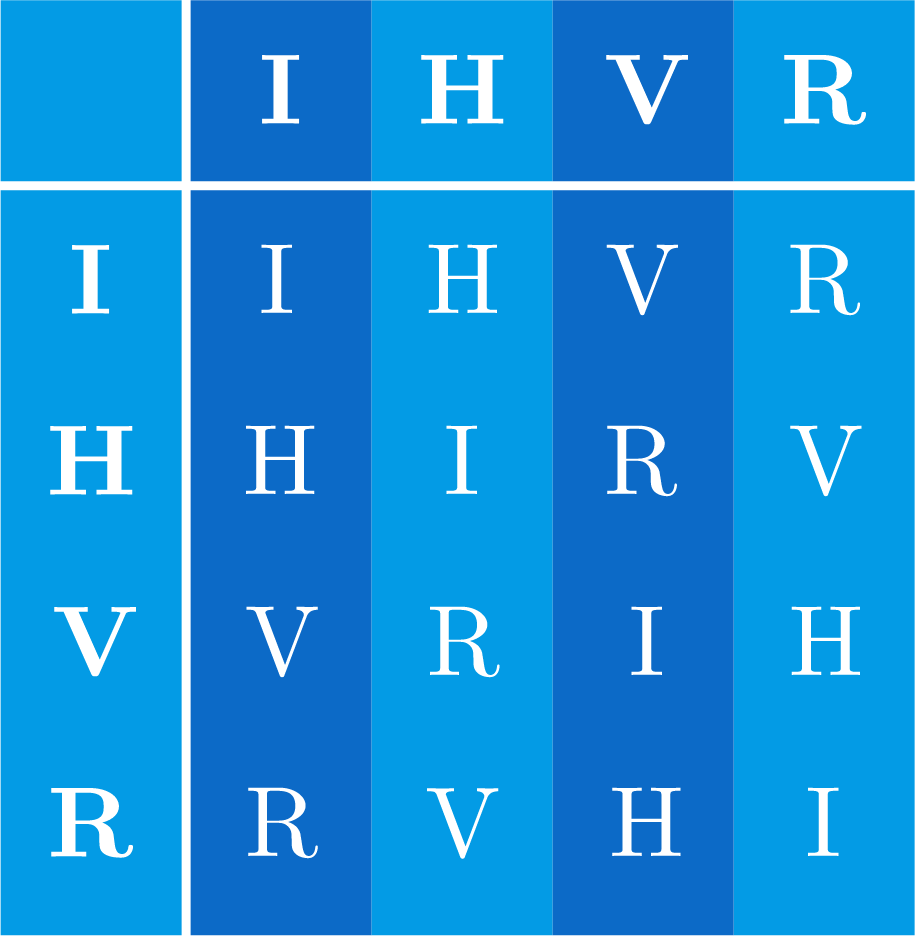This table completely describes all products of the four symmetries of the letter I.

# Combining Symmetries

Let’s stop for a moment and do something completely different. (At least, it will seem completely different at first…)

Suppose we only have two numbers, 0 and 1. Suppose we consider addition “mod 2” on these numbers, which means that it works exactly as normal, except that 1 + 1 = 0. We could make a little table for this operation: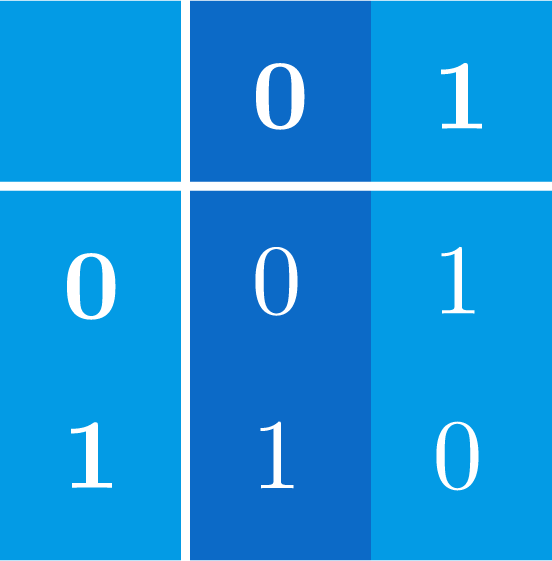Now, let’s do the same thing to ordered pairs of 0’s and 1’s, like $(0,1)$ and $(1,0).$ How do you add two such pairs? Just add the $x$-coordinates and $y$-coordinates separately. (Remember that 1 + 1 is still 0.) So $(0,1) + (1,0) = (1,1),$ and $(1,1) + (1,1) = (0,0).$

Here’s what the “addition table” for this operation on pairs looks like: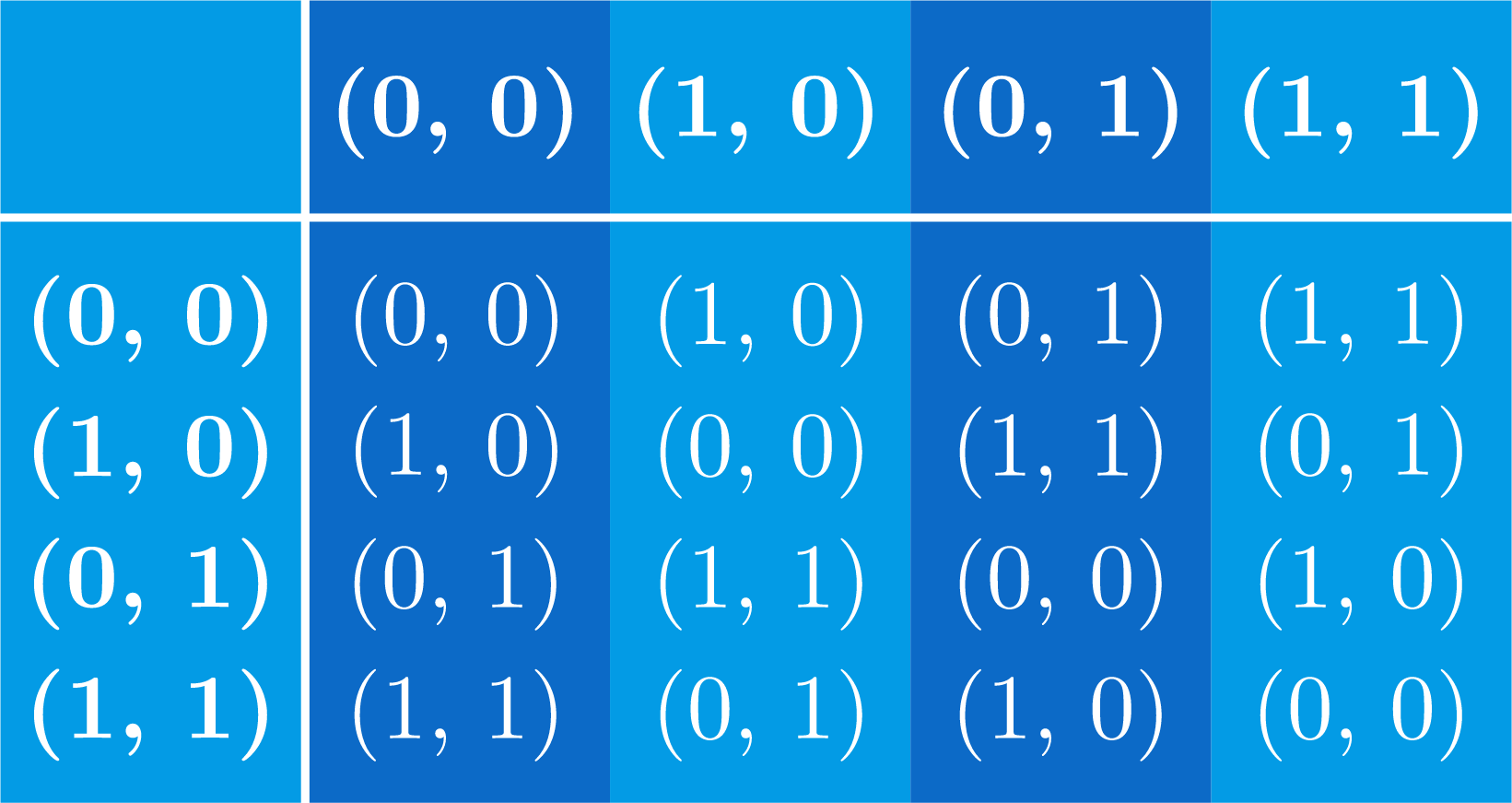Do you see what the connection is between this addition table and the multiplication table we made for the letter I? Think about it, stare at both tables, and then turn to the next page for the answer…

# Combining Symmetries

To see the connection, suppose we rename the four ordered pairs. Let’s denote $(0,0)$ by $i,$ $(1,0)$ by $a,$ $(0,1)$ by $b$ and $(1,1)$ by $c$. Then the addition table becomes: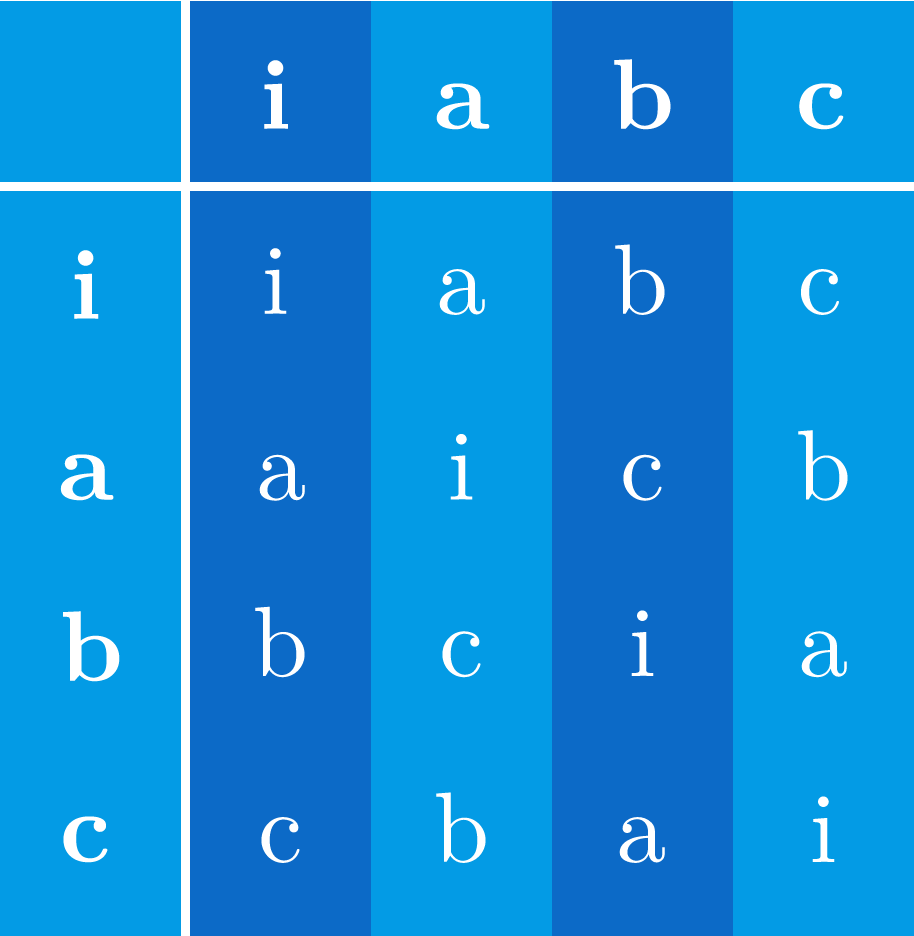This is the same table as the symmetries for the letter I, just with different variable names! In other words, the algebraic structure of the symmetries of the letter I is the same as the algebraic structure of ordered pairs of 0’s and 1’s under mod 2 addition. There is some underlying mathematical object that represents this structure… and we will call that object a group.

This particular table will be represented by something called the Klein four-group. We will encounter it again, along with many other interesting examples.

# Combining Symmetries

×